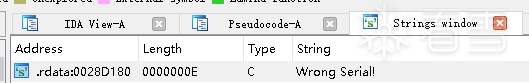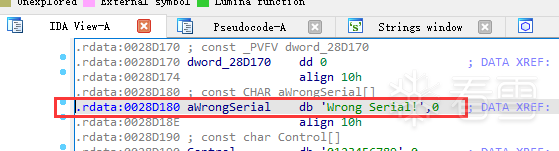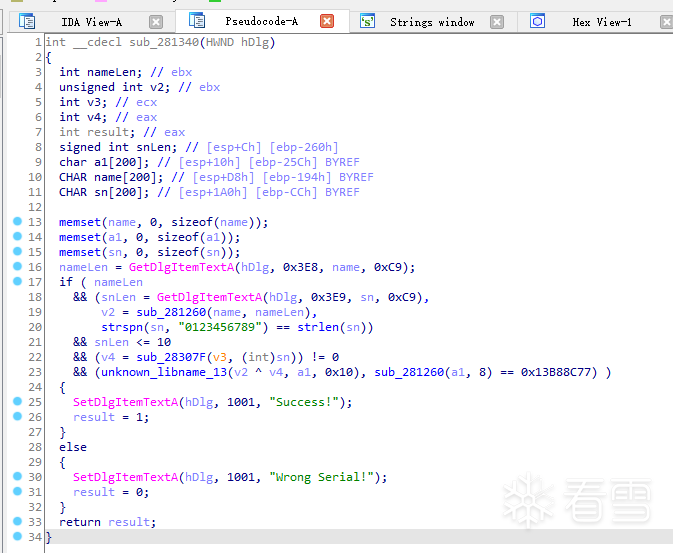2021-11-15 13:54
1714

IDA

# 【2、推导过程】

## 2.1、判断公式

（1）、F(用户名) xor HEX(序列号) = 52A1ED5A
（2）、G(52A1ED5A) = 13B88C77

## 2.2、经过动态跟踪：

F("01F845C5B7C52E56") = 744E1CC7
F("KCTF") = 5EE54F4C

## 2.3、最终要求出：

HEX(序列号) = 5EE54F4C xor 52A1ED5A = 0C44A216

# 【3、分析过程】

## 3.1、找到 字符串## 3.2、找到 关键代码## 3.3、分析 代码

v2 = sub_281260(name, nameLen)

strspn(sn, "0123456789") == strlen(sn)

C 库函数 size_t strspn(const char str1, const char str2) 检索字符串 str1 中第一个不在字符串 str2 中出现的字符下标。

snLen <= 10

v4 = sub_28307F(v3, (int)sn)

unknown_libname_13(v2 ^ v4, a1, 0x10)

a1 = v2 ^ v4

sub_281260(a1, 8) == 0x13B88C77)

## 3.4、调试 与 分析

（1）、用正确的用户名和序列号跟踪
sub_281260(a1, 8) == 0x13B88C77)
a1 = 52A1ED5A

v4 = sub_28307F(v3, (int)sn)

v4 = sub_28307F((int)sn)
sn = "653259165"
v4 = 26EFF19D

（2）、用正确的用户名KCTF和任意的序列号跟踪
v2 = sub_281260(name, nameLen)
v2 = 5EE54F4C

（3）、计算 v4
v2 = a1 ^ v4 => v4 = a1 ^ v2 = 52A1ED5A xor 5EE54F4C = 0C44A216

（4）、计算序列号
INT(0C44A216) = 205824534

[2023春季班]2023，新的征程，脱壳机更新、iOS/eBPF、赠送云手机套装！一块裸板虚拟化五个容器云手机！3月25日起同时上调价格并赠送新设备！